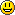# Welcome

Mathwiki is a self-study environment for students of computer science. It contains selection of math topics needed in their studies. The materials here include important definitions, general ideas of theory, lot of examples and different exercises with answers.

Solving the exercises is really important. Some of the exercises require a little programming – the easiest way to understand abstract theoretical concepts is through practical usage. If you can program it, you can understand it. Other exercises require analysis of algorithms. Theoretical exercises help the reader to understand different aspects of the theory.

For working with Mathwiki, note the following guide-lines.

• Do not use IE for Mathwiki - it will not work properly.
• Some lessons may take a minute or half for typesetting the math formulas.
• For fully understanding the lessons, do all the exercises in it.
• View the example solutions and answers only after you yourself have solved the exercise.
• Have fun working with MathwikiAdditional information about the environment (also user's guide) can be found HERE.